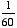index: click on a letter A B C D E F G H I J K L M N O P Q R S T U V W X Y Z A to Z index index: subject areas numbers & symbols sets, logic, proofs geometry algebra trigonometry advanced algebra & pre-calculus calculus advanced topics probability & statistics real world applications multimedia entrieswww.mathwords.com about mathwords website feedback

 Minute A unit of angle measure equal toof a degree. There are 60 minutes in one degree. Minutes are indicated using the ' symbol, so 12°45' means 12 degrees and 45 minutes, or 12.75 degrees.     See also Second